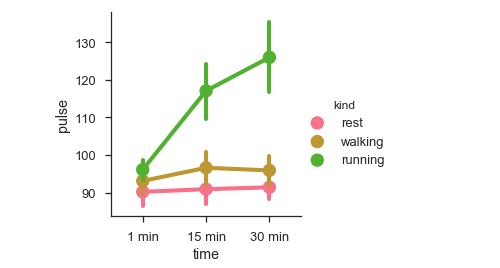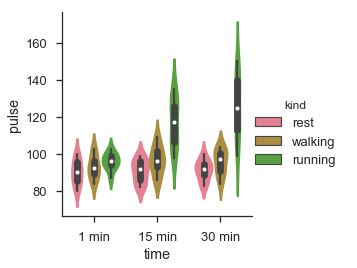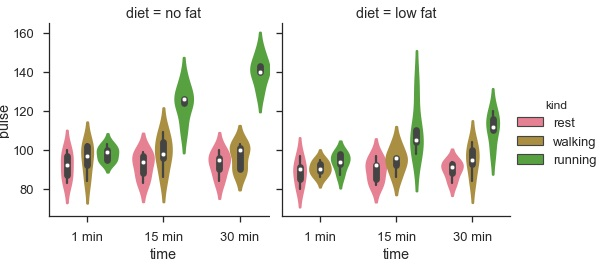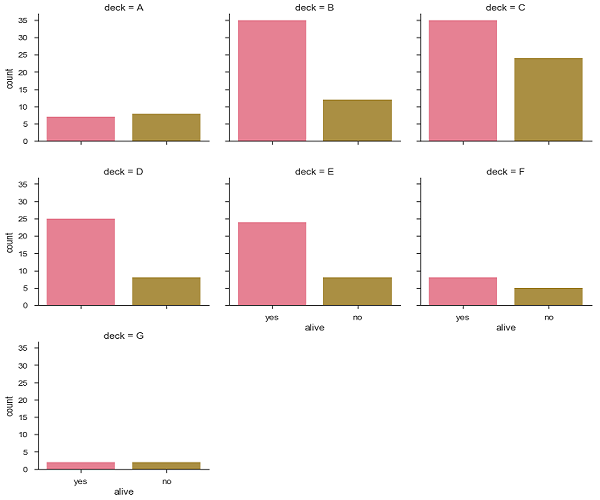# Seaborn - Multi Panel Categorical Plots

#### Data Visualization using MatPlotLib & Seaborn

11 Lectures 4 hours

#### Seaborn with Python

11 Lectures 2.5 hours

#### Data Visualization in Python Using Seaborn Library

20 Lectures 2 hours

Categorical data can we visualized using two plots, you can either use the functions pointplot(), or the higher-level function factorplot().

## Factorplot

Factorplot draws a categorical plot on a FacetGrid. Using ‘kind’ parameter we can choose the plot like boxplot, violinplot, barplot and stripplot. FacetGrid uses pointplot by default.

### Example

```import pandas as pd
import seaborn as sb
from matplotlib import pyplot as plt
sb.factorplot(x = "time", y = pulse", hue = "kind",data = df);
plt.show()
```

### OutputWe can use different plot to visualize the same data using the kind parameter.

### Example

```import pandas as pd
import seaborn as sb
from matplotlib import pyplot as plt
sb.factorplot(x = "time", y = "pulse", hue = "kind", kind = 'violin',data = df);
plt.show()
```

### OutputIn factorplot, the data is plotted on a facet grid.

## What is Facet Grid?

Facet grid forms a matrix of panels defined by row and column by dividing the variables. Due of panels, a single plot looks like multiple plots. It is very helpful to analyze all combinations in two discrete variables.

Let us visualize the above the definition with an example

### Example

```import pandas as pd
import seaborn as sb
from matplotlib import pyplot as plt
sb.factorplot(x = "time", y = "pulse", hue = "kind", kind = 'violin', col = "diet", data = df);
plt.show()
```

### OutputThe advantage of using Facet is, we can input another variable into the plot. The above plot is divided into two plots based on a third variable called ‘diet’ using the ‘col’ parameter.

We can make many column facets and align them with the rows of the grid −

### Example

```import pandas as pd
import seaborn as sb
from matplotlib import pyplot as plt